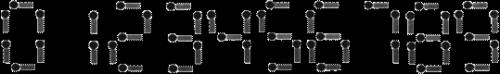# P1028 数的计算 传播

## 题目描述

1. 不作任何处理;
2. 在它的左边加上一个自然数,但该自然数不能超过原数的一半;
3. 加上数后,继续按此规则进行处理,直到不能再加自然数为止.

## Code $O(n)$

### 递推优化 $O(n)+O(n)$

#### 就地传播 $O(n)+O(0.25n)$#### 就地+队列 $O(0.5n)+O(0.25n)$

sum只要求到n/2就可以求出f[n]sum只要求到n/4就可以求出f[n/2]3+7+12=22

3+7+19=29

7+12+19=38

3+12+19=34

# P1149 火柴棒等式 模拟+打表

## 题目描述1. 加号与等号各自需要两根火柴棍
2. 如果A≠B，则A+B=C与B+A=C视为不同的等式(A,B,C>=0)
3. n根火柴棍必须全部用上

## Code $O(n^2)$

C++ STL MultiSet类成员函数介绍及具体用法示例

OJ程序：

# *P1217 [USACO1.5]回文质数 Prime Palindromes 素数筛法+回文模拟

## 说明

Hint 1: Generate the palindromes and see if they are prime.

Hint 2: Generate palindromes by combining digits properly. You might need more than one of the loops like below.

USACO Training Section 1.5

## Code $\approx O(n)$

### Force $O((b-a)logn)$

#### 改进is_prime 66% $O((b-a)\sqrt n)$

for循环条件改为i*i<=number即可。（避免重复计算，比如对于62,33,2只须判断一次即可）

### 埃氏筛法 88% $O(nloglogn)+O(n)$

Force中的尾递归可改写为迭代形式。• 初始化：默认所有数为素数（也可以附加设定01不是素数）
• i=2开始，遍历最近的素数（到根号n为止）
• j=i*i开始，步长为i，遍历并标记合数

*复杂度$O(nloglogn)​$的证明：（证了等于没证

• 本质是对数列和$S_n​$的渐进估计：（p取不大于n的所有素数）

### 欧拉筛法 88% $\approx O(n)+O(n+\frac{n}{logn})$

• 枚举每一个数i
• i是素数，先存入素数表p_numbers（显然素数表单增）
• J遍历素数表（生成关于i*J的乘法元集，并筛掉）
• 先筛掉i*J默认筛
• i%J==0（即i*J=A*J*J
• J’>J是素数表中任意一个比J更大的素数
• 接下来要筛的任何一个对象i*J'=(A*J)*J'=(A*J')*J，都会在之后被一个比i更大的i'=(A*J')默认筛掉
• 而且只要被筛对象合法，i'=(A*J')<i*J'肯定会被遍历到
• 而且能保证不会重复筛
• 假设i'*J'之前被i筛过，那么必然有i'*J'=(i*J)*J'=i*(J*J')，而(J*J')是合数，矛盾
• 显然无须继续遍历，提前退出即可

printf("%.2f", (double)clock()/CLOCKS_PER_SEC);可以用来显示运行时间。

p_numbers[MAX_N]MAX_N最小可以换为MAX_N/ln(MAX_N)。（$\pi(x)=\cfrac{x}{lnx}​$）

### 回文数整流

• Eratosthenes(b>10000000 ? 10000000: b);
• Euler(b>10000000 ? 10000000: b);
• 两个都可以擦边过。（耗时很接近）

### 区间素数筛法 $O(\sqrt n+(b-a)loglogn)+O(\sqrt n+(b-a))$

P1835 素数密度_NOI导刊2011提高（04）

### 打表 $O(x)+O(x)$

x是所有回文质数的数量。

OJ程序：

### 二分打表 $O(logx)+O(x)$

c++貌似有标准函数lower_bound()。。

### 回文数生成【正解】

POJ 3247:回文素数—外表甜美内心却极为暴力的萝莉题

0%